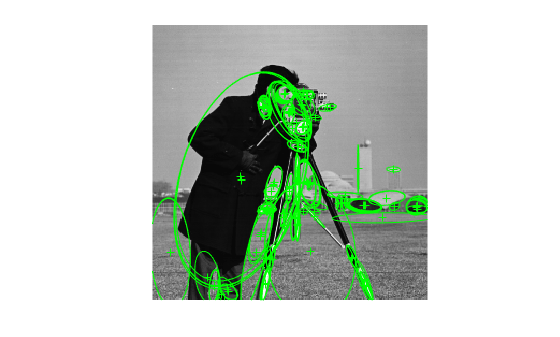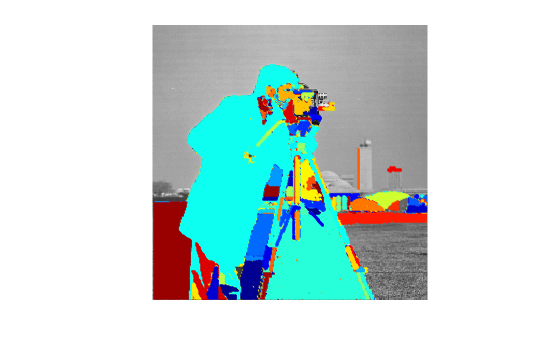Documentation

# plot

Plot MSER regions

## Syntax

``plot(points)``
``plot(points,ax)``
``plot(points,ax,Name,Value)``

## Description

example

````plot(points)` plots points in the current axis.```
````plot(points,ax)` plots points in the specified axis.```
````plot(points,ax,Name,Value)` sets properties using one or more name-value pairs. Enclose each property name in quotes. For example, `plot('ShowOrientation',true)````

## Examples

collapse all

Extract MSER features and plot the regions.

Read image and extract MSER features.

```I = imread('cameraman.tif'); regions = detectMSERFeatures(I); imshow(I); hold on; plot(regions);```Plot MSER Regions.

```figure; imshow(I); hold on; plot(regions,'showPixelList',true,'showEllipses',false); hold off;```## Input Arguments

collapse all

Points, specified as a points object. The object contains information about the feature points detected in the 2-D grayscale input image.

Handle to use for display. You can set the handle using `gca`.

### Name-Value Pair Arguments

Example: `'ShowOrientation'`,`'true'`

Display ellipsis around feature, specified as `true` or `false`. When you set this value to `true`, the object draws an ellipse with the same 2nd order moments as the region. When you set this value to `false`, only the ellipses centers are plotted.

Display feature point orientation, specified as `true` or `false`. When you set this value to `true`, the object draws a line corresponding to the point's orientation. The object draws the line from the feature point location to the edge of the circle, indicating the scale.

Display regions using JET colormap, specified as `true` or `false`.